Courses

# JEE(MAIN) Mathematics Mock Test - 8

## 30 Questions MCQ Test JEE Main Mock Test Series 2020 & Previous Year Papers | JEE(MAIN) Mathematics Mock Test - 8

Description
This mock test of JEE(MAIN) Mathematics Mock Test - 8 for JEE helps you for every JEE entrance exam. This contains 30 Multiple Choice Questions for JEE JEE(MAIN) Mathematics Mock Test - 8 (mcq) to study with solutions a complete question bank. The solved questions answers in this JEE(MAIN) Mathematics Mock Test - 8 quiz give you a good mix of easy questions and tough questions. JEE students definitely take this JEE(MAIN) Mathematics Mock Test - 8 exercise for a better result in the exam. You can find other JEE(MAIN) Mathematics Mock Test - 8 extra questions, long questions & short questions for JEE on EduRev as well by searching above.
QUESTION: 1

Solution:
QUESTION: 2

Solution:
QUESTION: 3

### The area, in square unit, of the region bounded by the curve x2 = 4y , the line x = 2 and the x-axis is

Solution:
QUESTION: 4

The radical axis of the circles, belongs to the coaxial system of circles whose limiting points are (1,3) and (2,6) is

Solution:
QUESTION: 5

If y=sin((1+x2)/(1-x2)), (dy/dx)=

Solution:
QUESTION: 6

If z₁ and z₂ are any two complex numbers then |z₁ + z₂|2 + |z₁ - z₂|2 =

Solution:
QUESTION: 7

If the line 2x - y + k = 0 is a diameter of the circle x2 + y2 + 6x -6y + 5 =0, then k is equal to

Solution:
QUESTION: 8
The solution of the differential equation cos x sin y dx + sin x cos y dy =0 is
Solution:
QUESTION: 9

The complex equation |z + 1 − i| = |z + i − 1| represents a

Solution:
QUESTION: 10
The minimum value of sinx + cosx is
Solution:
QUESTION: 11

In the following question, a Statement of Assertion (A) is given followed by a corresponding Reason (R) just below it. Read the Statements carefully and mark the correct answer-
Assertion (A): Three vectors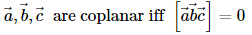Reason (R): The coplanar vectors are linearly independent

Solution:
QUESTION: 12

Which of the following is a Contradiction ?

Solution:
QUESTION: 13

In the following question, a Statement-1 is given followed by a corresponding Statement-2 just below it. Read the statements carefully and mark the correct answer-
Consider the system of equations
x - 2y + 3z = -1
- x + y - 2z = k
x - 3y + 4z = 1
Statement-1:
The system of equations has no solution for k ≠3.
Statement-2:
The determinant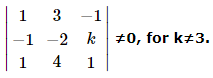Solution:
QUESTION: 14
If the traces of the matrices A and B are 20 and -8, then the trace of (A + B)=
Solution:
QUESTION: 15

Twelve students compete for a race. The number of ways in which first three prizes can be taken is

Solution:
QUESTION: 16

The tangents at the points a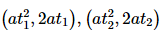on the parabola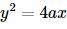are at right angles if

Solution:
QUESTION: 17
If the coefficient of correlation between x and y is 0.28, covariance between x and y is 7.6, and the variance of x is 9, then the standard deviation of the y series is
Solution:
QUESTION: 18

Points (0,0), (2,-1) and (9,2) are vertices of a triangle, then cosB =

Solution:
QUESTION: 19
8 coins are tossed simultaneously. The probability of getting at least 6 heads is
Solution:
QUESTION: 20

The angle between the lines (x - 1)/1 = (y - 1)/1 = (z - 1)/2 and (x - 1)/(- √3 - 1) = (y - 1)/(√3 - 1) = (z - 1)/4 is

Solution:
QUESTION: 21

The straight line x + y = a will be a tangent to the ellipse x2/9 + y2/16 = 1 if a =

Solution:
QUESTION: 22
The value of cosA-sinA when A=(5π/4), is
Solution:
QUESTION: 23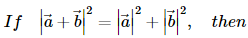Solution:
QUESTION: 24

The projection of a along b is

Solution:
QUESTION: 25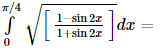Solution:
QUESTION: 26

In the following question, a Statement of Assertion (A) is given followed by a corresponding Reason (R) just below it. Read the Statements carefully and mark the correct answer-
Assertion(A): If arg(z) < 0, then arg (-z)-arg(z) = -π.
Reason(R): The complex number z satisfying
|z-1| = |z-3| = |z-i| is 2 + 2i.

Solution:
QUESTION: 27

If |A| represents the determinant of a square matrix of order 3 then (-2A)=

Solution:
QUESTION: 28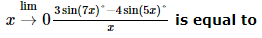Solution: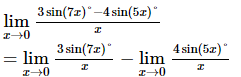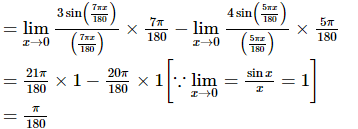QUESTION: 29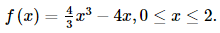Then the global minimum value of the function is

Solution: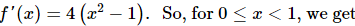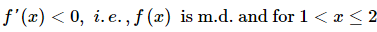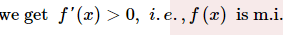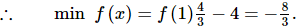QUESTION: 30

Consider an infinite series with first term 'a' and common ration 'r'. If it sum is 4 and the second term is 3/4, then

Solution: